Courses

Test: Covalent And Coordinate Bonding

28 Questions MCQ Test Chemistry for JEE | Test: Covalent And Coordinate Bonding

Description
Attempt Test: Covalent And Coordinate Bonding | 28 questions in 28 minutes | Mock test for JEE preparation | Free important questions MCQ to study Chemistry for JEE for JEE Exam | Download free PDF with solutions
QUESTION: 1

Direction (Q. Nos. 1-15) This section contains 15 multiple choice questions. Each question has four choices (a), (b), (c) and (d), out of which ONLY ONE option is correct. Q. By Lewis structure NO has following fact

Solution: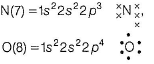To complete octet, N shares 2 electrons and oxygen shares 3 electrons.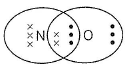Thus, (a) is correct.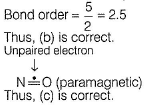QUESTION: 2

Lewis structure of HCI, H2O and NH3 are

Solution: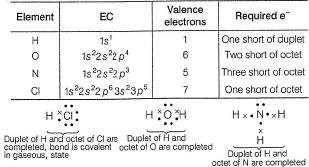QUESTION: 3

The maximum covalency is equal to :

Solution:

The maximum covalency is total no. of  unpaired electron in ground state or in excited state which can be used for bond formation.

QUESTION: 4

Which of the following has incomplete octet?

Solution: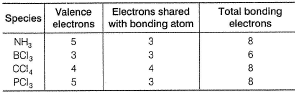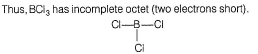QUESTION: 5

Expansion of octet can not take place in

Solution:

N(7) = 1s22s22p3
Nitrogen does not have (2d) orbitals. Thus, (more than 8) electrons cannot be accommodated in the second orbit.

QUESTION: 6

Which of the following is electron deficient?

Solution: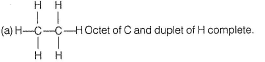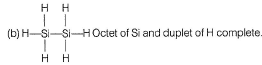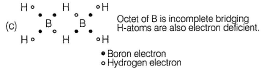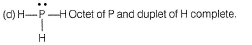QUESTION: 7

Based on valence bond theory, types of bonding in H2O are

Solution: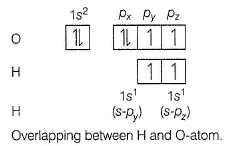QUESTION: 8

Bond order of (B— H) x and y bonds in diborane are respectively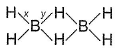Solution:

Based on structure: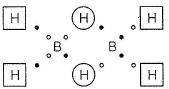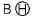bond has one electron; bond order y = 0.5.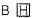bond has two electrons; bond order x = 1.0.

QUESTION: 9

Considering x-axis as the internuclear axis, which out of the following will not form sigma bond.

Solution: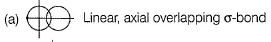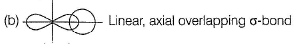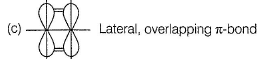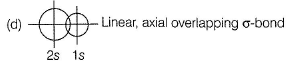QUESTION: 10

In which of the following molecules would you expect the N to N bond to be shortest ?

Solution: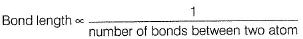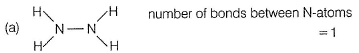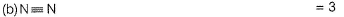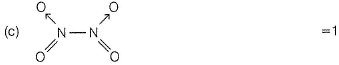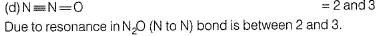QUESTION: 11

Which of these molecules have non-bonding electron pairs on the central atom?

I. SF4
II. ICI3
III. SO2
IV. NH3
V. SiF4

Solution:

Nonbonding electron pairs means lonepair of electrons.​

• SF4​ molecule has one non-bonding electron pair on the central atom Sulfur.
• ICl3​ molecule has two non-bonding electron pairs on the central atom Iodine.
• SO2​ molecule has one non-bonding electron pair on the central atom Sulfur.

QUESTION: 12

HCN and HNC molecules have equal number of

Solution: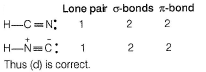QUESTION: 13

When a chemical bond is formed, there is decrease in

Solution:

When two atoms approach to form a bond, there is decrease in potential energy (PE). After bond formation, at a minimum distance (called bond length). PE further increases.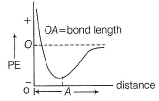QUESTION: 14

Mean bond enthalpy of different bonds are given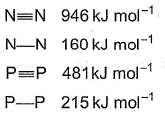Out of the given pairs, which compound is more stable than the other?

Solution: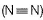)bond enthalpy >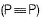)bond enthalpy hence,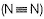is stable.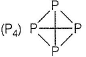Bond enthalpy of six (P— P) bond = 6 x 215 = 1290 k j mol-1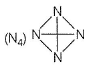Bond enthalpy of six (N — N) bond = 6 x 160
= 960 kJ mol-1
Thus, P4 is more stable than N4.

QUESTION: 15

Covalency of carbon in CO is three because

Solution: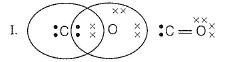In structure I, octet of oxygen is complete but octet of carbon is incomplete. Hence, carbon is an acceptor of an electron pair from oxygen. Thus, covalency of carbon is three in CO.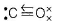*Multiple options can be correct
QUESTION: 16

Q. Which of the following species contain at least one atom that violates the octet rule?

Solution:

(a) Octet of Cl expanded to (9).
(b) Octet Xe expanded to (10).
(c) Octet of P expanded to (10).

*Multiple options can be correct
QUESTION: 17

Select the correct statement(s) about NO2.

Solution: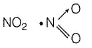Due to unpaired electron paramagnetic, N-atom in NO2 is electron deficie nt thus, to complete octet, dimer is formed.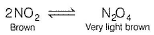In N2O4 formation, each N-atom gets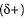charge. Dipole-dipole repulsion increases (N— N) bond length which is larger than (N— N)covalent bond.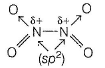Thus, (a), (b), (c) and (d) are correct.

*Multiple options can be correct
QUESTION: 18

Which of the following is/are correct statement(s)?

Solution:

Incorrect (b)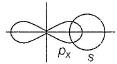two sp-hybrid orbitals are along x-axis.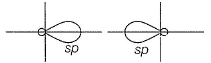*Multiple options can be correct
QUESTION: 19

Electron-seeking species are called electrophiles. Which of the following sets consists of electrophiles only?

Solution:

AICI3-Octet of Al is incomplete; thus electrophile.
SO3—It has positive charge on sulphur due to resonance.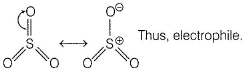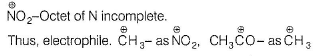*Multiple options can be correct
QUESTION: 20

Ratio of σ and π-bonds is equal to 1 in

Solution: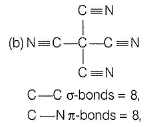Tetracyanomethane, where σ bond =8, ratio = 1, π bond = 8.

Carbon dioxide, O=C=O

σ = 2, π = 2

Ratio = 1.

QUESTION: 21

Direction (Q. Nos. 21-25) This section contains  a paragraph, wach describing theory, experiments, data etc. three Questions related to paragraph have been given.Each question have only one correct answer among the four given options (a),(b),(c),(d)
Passage I

Electronegativity values (EN) of elements have been given (in Pauling’s scale)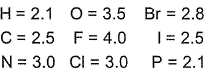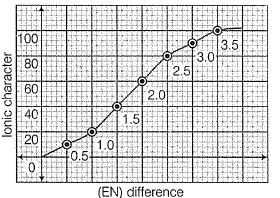Graph shows variation of percentage ionic character with (EN) difference of two elements.
Q. Which of the following bonds is most polar?

Solution: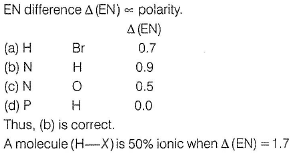QUESTION: 22

Passage I

Electronegativity values (EN) of elements have been given (in Pauling’s scale)Graph shows variation of percentage ionic character with (EN) difference of two elements.
Q. A molecule o f the type H— X is 50% ionic when EN difference is

Solution:QUESTION: 23

Passage II

Consider the following structure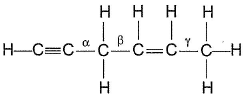Q. Shortest (C— H) bond is

Solution:

Greater the electronegativity of carbon atom
(sp3 < sp2 < sp)
Greater the attraction for (C— H) bonding pair hence, shorter the (C— H)bond.

QUESTION: 24

Passage II

Consider the following structureQ. Shortest (carbon-carbon) bond is

Solution: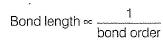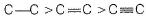QUESTION: 25

Which among the following chemical bond were described by Kossel and Lewis?

Solution:

Both Ionic and Covalent bond arise from the tendency of atoms to attain stable configuration of electrons.

QUESTION: 26

Direction (Q. Nos. 26) Choice for the correct combination of elements from coloumn I and Coloumn II are given as option (a), (b), (c) and (d) out of which one is correct.
Match the species in Column I with their properties in Column II.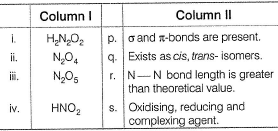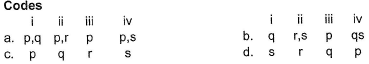Solution: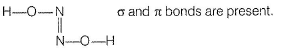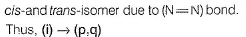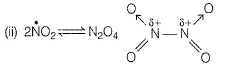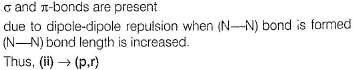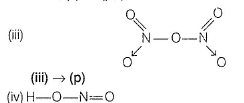α and π bonds exist.
Oxidation number of N = + 3 it can be increased to +5 (maximum) and decreased to -3 (minimum) HNO2 is also a ’ligand.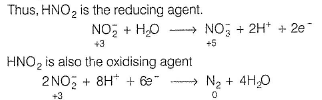HNO2 is also the complexing agent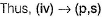*Answer can only contain numeric values
QUESTION: 27

Direction (Q. Nos. 27 and 28) This section contains 2 question. when worked out will result in an integer from 0 to 9 (both inclusive)

Q. In acidic medium,H2O2 changes Cr2O72- to CrO5 which has two (-O-O-) bonds. Oxidation state of Cr in CrO3 is

Solution:

When H2O2 is added to an acidified solution of a dichromate, Cr2O72-, aO deep blue coloured complex, chromic peroxide, CrO5 [ or CrO(O2)2] is formed.
Cr2O72- +2H++4H2O2 ---> 2CrO(O2)2 +5H2O
This deep blue coloured complex.

Oxidation state of Cr is +6 due to the presence of two peroxide linkages which can be calculated as Cr peroxide normal
x+(-1)4+(-2) =0
x-6 =0
x=+6

*Answer can only contain numeric values
QUESTION: 28

K2Cr2O7 is an oxidising agent in acidic medium. How many O-atoms are directly linked to each Cr-atom?

Solution: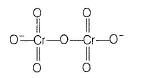There are 4 atoms that are directly linked to each Cr atom.Use Code STAYHOME200 and get INR 200 additional OFF Use Coupon Code

Track your progress, build streaks, highlight & save important lessons and more!

Similar Content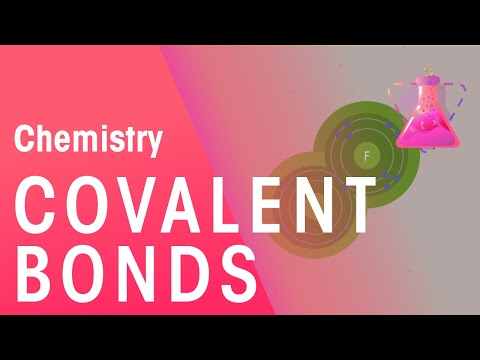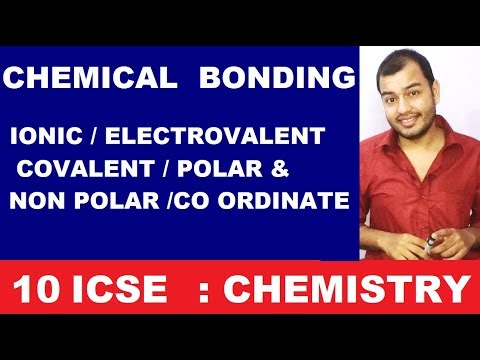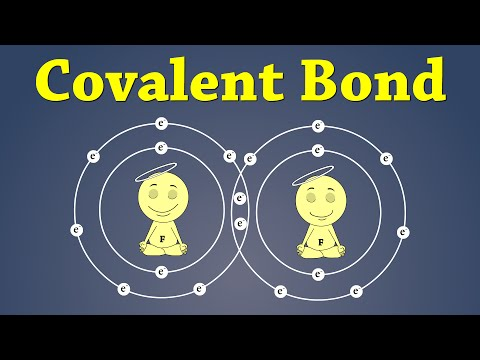Related tests